Core Java - Interview Questions and Answers for 'Object equality' | Search Java Interview Question - javasearch.buggybread.com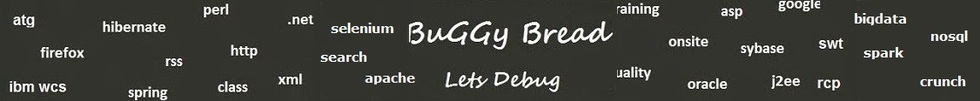Search Interview Questions3335 questions in repository.There are more than 200 unanswered questions.Have a video suggestion.
Click Correct / Improve and please let us know.
Label / Company      Label / Company / TextSubmit Question

Core Java - Interview Questions and Answers for 'Object equality' - 9 question(s) found - Order By Newest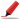Very frequently asked. Among first few questions in almost all interviews. Among Top 5 frequently asked questions. Frequently asked in Indian service companies (HCL,TCS,Infosys,Capgemini etc based on multiple feedback )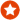Q1. Difference between == and .equals() ?Core Java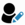Anonymous
Ans. "equals" is the method of object class which is supposed to be overridden to check object equality, whereas "==" operator evaluate to see if the object handlers on the left and right are pointing to the same object in memory.

x.equals(y) means the references x and y are holding objects that are equal. x==y means that the references x and y have same object.

Sample code:

String x = new String("str");
String y = new String("str");

System.out.println(x == y); // prints false
System.out.println(x.equals(y)); // prints true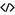Sample Code for equalsHelp us improve. Please let us know the company, where you were asked this question :LikeDiscussCorrect / Improvejava   string comparison   string   object class   ==    equals   object equality  operator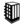Asked in 289 Companies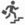basic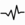frequent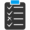Try 6 Question(s) TestQ2. What will be the output ?Integer a = 10, b =10;Integer c = 10, d = 1000;System.out.println(a == b);System.out.println(c ==d);Core Javaadmin info@buggybread.com
Ans. truefalseHelp us improve. Please let us know the company, where you were asked this question :LikeDiscussCorrect / Improvejava   coding   equality   object equalityQ3. What will the following code print ?

Integer a = 100, b =100;
Integer c = 1000, d = 1000;
System.out.println(a == b);
System.out.println(c ==d);
Core JavaAns. false
falseHelp us improve. Please let us know the company, where you were asked this question :LikeDiscussCorrect / Improveobject equality  code  codingfrequentQ4. What is the difference between = and == in Java ?Core Java2016-09-02 08:12:45Ans. = is the assignment operator that assigns the result of the expression on the right to the variable on the left, whereas

== is the operator to check object equality to see if the reference on left and right are pointing to the same object. For primitive types, its used to check if both variables holds the same value.Help us improve. Please let us know the company, where you were asked this question :LikeDiscussCorrect / Improve=  ==  assignment operator  object equality  difference betweenBasicQ5. How is == operator different for objects and primitive types ? Core Java2016-09-02 08:14:44Ans. For objects or references, == operator check if the reference on left and right points to the same object.

For primitive types or variables, == operator check if the variable on left and right holds the same value.Help us improve. Please let us know the company, where you were asked this question :LikeDiscussCorrect / Improve==  object equality  operatorBasicQ6. What will be the output of following code

Integer x = 1;
Integer y = 2;
System.out.println(x == y);

What if you change 1 to "1" and Integer to String?
Core Java2017-05-07 19:30:13Ans. It will print "true" with integers as well as strings. The reason is "Integer constant pool" and "String pool"

String pool maintains pool of string literals. When a string literal is used for the first time, a new string object is created and is added to the pool. Upon it's subsequent usage , the reference for the same object is returned. Similarly java uses integer constant pool.Help us improve. Please let us know the company, where you were asked this question :LikeDiscussCorrect / Improvestring pool  object equality  ==Q7. What will be the output of following code

class TestMain {
public static void main(String[] args) {
String s1 = "ravi";
String s2 = new String("ravi");
Integer i = 10;
Integer a1 = new Integer("10");
System.out.println(s1 == s1);
System.out.println(i == a1);
}
}
Core Java2017-07-17 08:07:19Ans. false
falseHelp us improve. Please let us know the company, where you were asked this question :LikeDiscussCorrect / Improveobject equality  ==  coding  codeAsked in 1 CompaniesQ8. What will be the output of following ? String str1 = new String("String1"); String str2 = new String("String1"); System.out.print(str1 == str2); System.out.print(str1.equals(str2)); str1 = str2; System.out.print(str1 == str2); Core Java
a. falsetruetrue
b. truetruetrue
c. truetruefalse
d. falsetruefalse

Ans.a. falsetruetrueQ9. What will be the output of following code ? String str1 = "String1"; String str2 = "String1"; System.out.print(str1 == str2); System.out.print(str1.equals(str2)); str1 = str2; System.out.print(str1 == str2); Core Java
a. falsetruetrue
b. falsefalsetrue
c. truetruetrue
d. falsefalsefalse

Ans.c. truetruetrue

Subscribe to Java News and Posts. Get latest updates and posts on Java from Buggybread.com